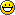## Tutorial: Create a Tower Defense Game in AS3 – Part 4

Written By MrSun at 8:04 am - Saturday, February 28th, 2009
Categories: Advanced Tutorials, All Tutorials, AS3, Flash, Game Development

### Step 4: Making Turrets Attack Enemies

Well, it’s now time to let the enemies we just created be destroyed. Let’s dig in, shall we?

First, we’ll define a single health variable for the Enemy. Just add this code to “Enemy.as” where we define all of the variables:

`public var health:int = 5;`

Next, we’re going to have to create a new class. We’ve done this many times before, so hopefully I don’t need to remind you how to do it. Just create a new ActionScript file and save it as “Bullet.as”. Now, we’re just going to have to place a bit of code into it:

```package { import flash.display.MovieClip; import flash.events.*; public class Bullet extends MovieClip { private var _root:*; //these two variables below must be set to public so that we can edit them outside the class public var target;//the target that this guy is moving towards public var damage:int;//how much damage this guy inflicts on the enemy   private var xSpeed:Number;//how fast it's moving horizontally private var ySpeed:Number;//how fast it's moving vertically private var maxSpeed:Number = 5;//how fast it can go public function Bullet() { addEventListener(Event.ADDED,beginClass);//this will run every time this guy is made addEventListener(Event.ENTER_FRAME,eFrame);//this will run every frame } private function beginClass(e:Event):void { _root = MovieClip(root);//setting the root   //drawing this guy (it'll be a small white circle) this.graphics.beginFill(0xFFFFFF); this.graphics.drawCircle(0,0,2); this.graphics.endFill(); } private function eFrame(e:Event):void { var yDist:Number=target.y+12.5 - this.y;//how far this guy is from the enemy (x) var xDist:Number=target.x+12.5 - this.x;//how far it is from the enemy (y) var angle:Number=Math.atan2(yDist,xDist);//the angle that it must move ySpeed=Math.sin(angle) * maxSpeed;//calculate how much it should move the enemy vertically xSpeed=Math.cos(angle) * maxSpeed;//calculate how much it should move the enemy horizontally //move the bullet towards the enemy this.x+= xSpeed; this.y+= ySpeed;   if(this.hitTestObject(target)){//if it touches the enemy target.health -= damage;//make it lose some health destroyThis();//and destroy this guy } if(target == null || _root.gameOver == true){//destroy it if game is over destroyThis(); } } public function destroyThis():void{ //this function will just remove this guy from the stage this.removeEventListener(Event.ENTER_FRAME, eFrame); MovieClip(this.parent).removeChild(this); } } }```

The next thing we need to do is define some variables for the Turret. Open up “Turret.as” and add in the following code where we define the variables:

```private var angle:Number; //the angle that the turret is currently rotated at private var radiansToDegrees:Number = 180/Math.PI;//this is needed for the rotation private var damage:int = 3;//how much damage this little baby can inflict private var range:int = 100;//how far away (in pixels) it can hit a target private var enTarget;//the current target that it's rotating towards private var cTime:int = 0;//how much time since a shot was fired by this turret private var reloadTime:int = 12;//how long it takes to fire another shot private var loaded:Boolean = true;//whether or not this turret can shoot```

Those are a lot of variables, aren’t they? Well, this is a pretty complex task, so we’re going to need all of them. So, brace yourself, for we are now going to jump into some code. Add this code to the eFrameEvents function in “Turret.as”:

```//FINDING THE NEAREST ENEMY WITHIN RANGE var distance:Number = range;//let's define a variable which will be how far the nearest enemy is enTarget = null;//right now, we don't have a target to shoot at for(var i:int=_root.enemyHolder.numChildren-1;i>=0;i--){//loop through the children in enemyHolder var cEnemy = _root.enemyHolder.getChildAt(i);//define a movieclip that will hold the current child //this simple formula with get us the distance of the current enemy if(Math.sqrt(Math.pow(cEnemy.y - y, 2) + Math.pow(cEnemy.x - x, 2)) < distance){ //if the selected enemy is close enough, then set it as the target enTarget = cEnemy; } } //ROTATING TOWARDS TARGET if(enTarget != null){//if we have a defined target //turn this baby towards it this.rotation = Math.atan2((enTarget.y-y), enTarget.x-x)/Math.PI*180 - 90; if(loaded){//if the turret is able to shoot loaded = false;//then make in unable to do it for a bit var newBullet:Bullet = new Bullet();//create a bullet //set the bullet's coordinates newBullet.x = this.x; newBullet.y = this.y; //set the bullet's target and damage newBullet.target = enTarget; newBullet.damage = damage; _root.addChild(newBullet);//add it to the stage } } //LOADING THE TURRET if(!loaded){//if it isn't loaded cTime ++;//then continue the time if(cTime == reloadTime){//if time has elapsed for long enough loaded = true;//load the turret cTime = 0;//and reset the time } }```

Now, if you test the movie out, the turrets should be shooting at those darn red dots. Now, we have to make those dots die! In the eFrameEvents() function in “Enemy.as”, add the following code:

```//remove this from stage when game is over if(_root.gameOver){ destroyThis(); }```

Pretty hot stuff, ain’t it? Well, this concludes the fourth installment of this tutorial. Join us next time when we make levels and have winning and losing scenarios!

#### Final Product

Source Files (Zipped)

eMVy:

If your enemies are not being destroyed then add this code to “Enemy.as”:

if(this.health <= 0)
{
destroyThis();
}

enstine:

I don’t get about the tutorials. The source file can’t be open by my macromedia flash 8!

Blobbie:

What is this script (Math.atan2) mean ?
and
Can you explain this script “if(Math.sqrt(Math.pow(cEnemy.y – y, 2) + Math.pow(cEnemy.x – x, 2)) < distance)"

cole:

Thank you for making these great tutorials, for most new stuff that i try i like to do it without a tutorial just to test my problem solving skills. but some times they give you a good look at how other people do things. Very helpful, thanks